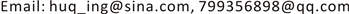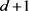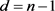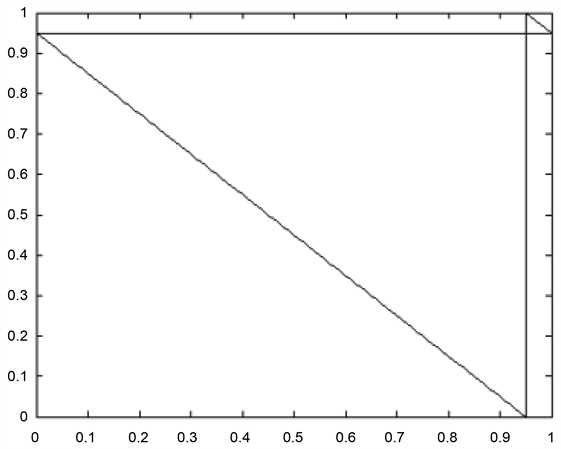﻿ 相依风险下的完全混合与随机变量和的最小Value-at-Risk Complete Mixability and Minimum Value-at-Risk of Sum of Dependent Variable

Statistics and Application
Vol.06 No.04(2017), Article ID:22516,8 pages
10.12677/SA.2017.64055

Complete Mixability and Minimum Value-at-Risk of Sum of Dependent Variable

Qing Hu, Yuxu Feng

School of Science, Beijing Technology and Business University, BeijingReceived: Oct. 8th, 2017; accepted: Oct. 23rd, 2017; published: Oct. 30th, 2017ABSTRACT

In general, the risk is in forms of portfolio in finance or in forms of the sum of insurance policy in insurance. And they are generally not independent and we do not know the correlation, and we call this correlation copula, of them. So we cannot research that using the method of investigating only one risk or regard random variables as independent. We need to consider the correlation of n random variables with given identical marginal distributions. In the quantitative risk management, the research about the sum of n random variables under uncertain correlation, especially focuses on the risk measure of the sum of n random variables. In this paper, we investigate $Va{R}_{\alpha }\left(S\right)$ (Value-at-Risk) and the copula that it can minimize the $Va{R}_{\alpha }\left(S\right)$ in a confident level. Then we get the copula of minimum of $Va{R}_{\alpha }\left(S\right)$ under monotone density. And this copula is that n random variables are complete mixable. Finally, this paper gets the minimum and maximum of $Va{R}_{\alpha }\left(S\right)$ at the situation of two dimensions. This result is also got under complete mixability.

Keywords:$Va{R}_{\alpha }\left(S\right)$ , Dependent Structure, Completely Mixable, Dependent Risk1. 引言

2. 风险度量

${\text{VaR}}_{\alpha }\left(S\right)={F}_{S}^{-1}\left(\alpha \right)=\mathrm{inf}\left\{s\in R:{F}_{S}\left(s\right)\ge \alpha \right\}$

${\text{ES}}_{\alpha }=E\left[{\left(S-{\text{VaR}}_{\alpha }\left(S\right)\right)}_{+}\right]$

${\text{TVaR}}_{\alpha }\left(S\right)=\frac{1}{1-\alpha }{\int }_{\alpha }^{1}\text{VaR}\left(S,t\right)\text{d}t$

${\text{CTE}}_{\alpha }\left(S\right)=E\left[S|S>{\text{VaR}}_{\alpha }\left(S\right)\right]$

3. 完全混合及相依结构

3.1. 完全混合

$n$ 是一个正整数，对于一个R上的概率分布F，如果存在 $n$ 个随机变量 ${X}_{1},\cdots ,{X}_{n}~F$ 使得 ${X}_{1}+\cdots +{X}_{n}$ 是一个常数，则称分布F是 $n$ 阶可完全混合的(或简称为 $n$ 阶可混合)。其中 $\left({X}_{1},\cdots ,{X}_{n}\right)$ 的联合分布成为一个 $n$ 阶可混合结构  ，(n-complete-mix)。

Sklar (1959)指出可以将随机变量的联合分布分解成边际分布和它们之间的相依结构(copula)，而变量之间的相依结构可以用copula来表达  ，下面给出copula函数的定义。

$\begin{array}{c}{C}_{Y}=P\left({F}_{{X}_{1}}\le {\mu }_{1},\cdots ,{F}_{{X}_{n}}\le {\mu }_{n}\right)\\ =P\left({X}_{1}\le {F}_{{X}_{1}}^{-1}\left({\mu }_{1}\right),\cdots ,{X}_{n}\le {F}_{{X}_{n}}^{-1}\left({\mu }_{n}\right)\right)\\ ={F}_{Y}\left({F}_{{X}_{1}}^{-1}\left({\mu }_{1}\right),,\cdots ,{Y}_{n}\le {F}_{{X}_{n}}^{-1}\left({\mu }_{n}\right)\right)\end{array}$

${\mu }_{j}={F}_{X}\left({x}_{j}\right),j=1,\cdots ,n$ ，我们可以得到

${F}_{X}\left({x}_{1},\cdots ,{x}_{n}\right)={C}_{X}\left({F}_{{X}_{1}}\left({x}_{1}\right),\cdots ,{F}_{{X}_{n}}\left({x}_{n}\right)\right)$

3.2. 同单调与反单调

3.2.1. 同单调

3.2.2. 反单调

3.3. 完全混合的性质和例子

3.3.1. 完全混合的性质

1) 完全混合仿射变换下的不变性

2) 完全混合分布角度的加性

3) 完全混合指数角度的加性

4) 完全混合的收敛性 

3.3.2. 完全混合的例子

1) 均匀分布的n(n ≥ 2)阶可混合性

a.s.，因此， $U\left[0,1\right]$ 是三阶可混合的，又因为它是2阶可混合的，所有大于1的正整数均可由2，3的组

2) 正态分布的n(n ≥ 2)阶可混合

$\mathrm{min}\text{Var}\left({X}_{1}+\cdots +{X}_{n}\right)$

4. 风险加和的VaR最小值问题

4.1. 问题相关定义和引理

4.1.1. 质量函数

4.1.2. 引理

${S}_{N}^{d}$ 上的一个质量函数A满足质量递减，且重心为0，则A一定是阶可混合的。其中 ${S}_{N}^{d}=\left\{-N,\cdots ,-1,0,1,\cdots ,dN\right\}$ 表示的是质量函数A的点集，${S}_{N}^{d}$ 中有 $\left(d+1\right)N+1$ 个点。

4.1.3. 推论

4.1.4 $minVa{R}_{\alpha }\left(S\right)$$maxVa{R}_{\alpha }\left(S\right)$

${X}_{1},\cdots ,{X}_{n}~\stackrel{˜}{F}$ (n为正整数)，密度函数为p(x)在区间 $\left[0,1\right]$ 上，在区间 $\left[0,1\right]$ 以外有 $p\left(x\right)=0$${X}_{1},\cdots ,{X}_{n}$ 相依结构未知， $S={X}_{1}+\cdots +{X}_{n}$ ，在置信度为 $\alpha$ 时，能否使得 ${\text{VaR}}_{\alpha }\left(S\right)$ 最小或最大，使得下式成立的相依结构是什么？

$\mathrm{min}{\text{VaR}}_{\alpha }\left(S\right)$$\mathrm{max}{\text{VaR}}_{\alpha }\left(S\right)$

$\mu \left(F\right)\le \frac{1}{2}$ 。当 $n=1$ 时满足条件的分布F是不存在的。当 $n=2$ 时，满足条件的分布只有均匀分布 $F=U\left[0,1\right]$

$n\ge 3$ 时，首先令 ${S}_{N}=\left\{-N/N,\left(-N+1\right),\cdots ,\left(dN-1\right)/N,dN/N\right\}$ ，在 $\left[-1,d\right]$$Y~F$ ，其均值为0，密度函数单调递减。令 ${\stackrel{¯}{F}}_{N}$ 为[NY]/N的分布函数，其中[NY]为NY的整数部分，{NY}表示NY的小数部分， ${\stackrel{^}{F}}_{N}$${S}_{N}$ 上的离散均匀分布的分布函数。由于

$\begin{array}{c}\mu \left({\stackrel{¯}{F}}_{N}\right)=E\left(\left[NY\right]/N\right)=E\left(\left(NY-\left\{NY\right\}\right)/N\right)=E\left(Y-\left\{NY\right\}/N\right)\\ =E\left(Y\right)-E\left(\left\{NY\right\}/N\right)=-E\left(\left\{NY\right\}/N\right)\end{array}$

$\frac{\left\{NY\right\}}{N}\in \left[-\frac{1}{N},0\right]$ ，所以其期望也在 $\left[-\frac{1}{N},0\right]$ 之间，因此 $\mu \left({\stackrel{¯}{F}}_{N}\right)\in \left[-\frac{1}{N},0\right]$

$\mu \left({\stackrel{^}{F}}_{N}\right)=\frac{d-1}{2}$

${\lambda }_{N}=\frac{-\mu \left({\stackrel{¯}{F}}_{N}\right)}{\frac{d-1}{2}-\mu \left({\stackrel{¯}{F}}_{N}\right)}$

$N\to \infty$ 时，根据完全混合的收敛性知， ${F}_{N}$ 依分布收敛于F，且F是 $d+1$ 阶可混合的。

$\mu \left(F\right)<\frac{1}{n}$ 时，无法构成完全混合结构，我们考虑以下的结构：

(a) 对每一个 $i=1,2,\cdots ,n$ ，给定 ${U}_{i}\in \left[0,c\right]$ ，我们有 ${U}_{j}=1-\left(n-1\right){U}_{i},\text{\hspace{0.17em}}\forall j\ne i$

(b) 对于所有的 ${U}_{i}\in \left[c,1-\left(n-1\right)c\right]$${F}^{-1}\left({U}_{1}\right)+\cdots +{F}^{-1}\left({U}_{n}\right)$ 是一个常数。

${Q}_{n}^{F}={Q}_{n}^{F}\left({c}_{n}\right)$ ，其中 ${c}_{n}$ 为copula ${Q}_{n}^{F}$ 满足(a) (b)条件存在的所有c中的最小值，并且 ${c}_{n}=0$ 当且仅当F是n阶完全混合的。定义 $H\left(x\right)={F}^{-1}\left(x\right)+\left(n-1\right){F}^{-1}\left(1-\left(n-1\right)x\right)$ ，则有

${c}_{n}=\mathrm{min}\left\{c\in \left[0,\frac{1}{n}\right]:{\int }_{c}^{\frac{1}{n}}H\left(t\right)\text{d}t\le \left(\frac{1}{n}-c\right)H\left(c\right)\right\}$

(c) 对每一个 $i=1,2,\cdots ,n$ ，给定 ${U}_{i}\in \left[1-c,1\right]$ ，我们有 ${U}_{j}=\left(n-1\right)\left(1-{U}_{i}\right),\text{\hspace{0.17em}}\forall j\ne i$

(d) 对于所有的 ${U}_{i}\in \left[\left(n-1\right)c,1-c\right]$${F}^{-1}\left({U}_{1}\right)+\cdots +{F}^{-1}\left({U}_{n}\right)$ 是一个常数。

${c}_{n}=\mathrm{min}\left\{c\in \left[0,\frac{1}{n}\right]:{\int }_{c}^{\frac{1}{n}}H\left(t\right)\text{d}t\ge \left(\frac{1}{n}-c\right)H\left(c\right)\right\}$

${F}^{-1}\left({U}_{1}\right)+\cdots +{F}^{-1}\left({U}_{n}\right)$ 是一个常数，因为 ${F}^{-1}\left({U}_{i}\right)$ 的密度单调递增，并且由 ${\int }_{c}^{\frac{1}{n}}H\left(t\right)\text{d}t\le \left(\frac{1}{n}-c\right)H\left(c\right)$ 可知，

$E\left({F}^{-1}\left({Z}_{1}\right)\right)\le {F}^{-1}\left(c\right)+\frac{n}{n-1}\left[{F}^{-1}\left(1-\left(n-1\right)c\right)-{F}^{-1}\left(c\right)\right]$

$y=\left\{\begin{array}{l}1+\alpha -x,\text{\hspace{0.17em}}\text{\hspace{0.17em}}\text{\hspace{0.17em}}x>\alpha \\ \alpha -x,\text{\hspace{0.17em}}\text{\hspace{0.17em}}\text{\hspace{0.17em}}\text{\hspace{0.17em}}\text{ }\text{\hspace{0.17em}}\text{\hspace{0.17em}}\text{\hspace{0.17em}}x\le \alpha \end{array}$Figure 1. The copula of anti-monotone with two variables

Complete Mixability and Minimum Value-at-Risk of Sum of Dependent Variable[J]. 统计学与应用, 2017, 06(04): 492-499. http://dx.doi.org/10.12677/SA.2017.64055

1. 1. R. 卡尔斯, M. 胡法兹, J. 达呐, M. 狄尼特. 现代精算风险理论—基于R [M]. 第二版. 北京: 科学出版社, 2016: 123-127.

2. 2. Bignozzi, V., Mao, T., Wang, B., et al. (2016) Diversification Limit of Quantiles under Dependence Uncertainty. Ex-tremes, 19, 143-170. https://doi.org/10.1007/s10687-016-0245-5

3. 3. Embrechts, P., Puccetti, G. and Rüschendorf, L. (2013) Model Uncertainty and VaR Aggregation. Journal of Banking & Finance, 37, 2750-2764. https://doi.org/10.1016/j.jbankfin.2013.03.014

4. 4. Puccetti, G., Wang, B. and Wang, R. (2013) Complete Mixability and Asymptotic Equivalence of Worst-Possible VaR and ES Estimates. Insurance Mathematics & Economics, 53, 821-828. https://doi.org/10.1016/j.insmatheco.2013.09.017

5. 5. 王良. VaR风险管理模型的理论与应用[D]: [硕士学位论文]. 大连: 东北财经大学, 2003.

6. 6. Wang, B. and Wang, R.D. (2011) The Complete Mixability and Convex Minimization Problems with Monotone Marginal Densities. Journal of Multivariate Analysis, 102, 1344-1360. https://doi.org/10.1016/j.jmva.2011.05.002

7. 7. 易文德. 随机变量间相依性度量的新方法[J]. 重庆文理学院学报(自然科学版), 2009, 28(5): 5-7, 14.

8. 8. 王彬. 随机变量的组合学[M]. 北京: 现代出版社, 2016: 17-29.

9. 9. Wang, R.D., Peng, L. and Yang, J.P. (2013) Bounds for the Sum of Dependent Risks and Worst Value-at-Risk with Monotone Marginal Densities. Finance and Stochastics, 17, 395-417. https://doi.org/10.1007/s00780-012-0200-5